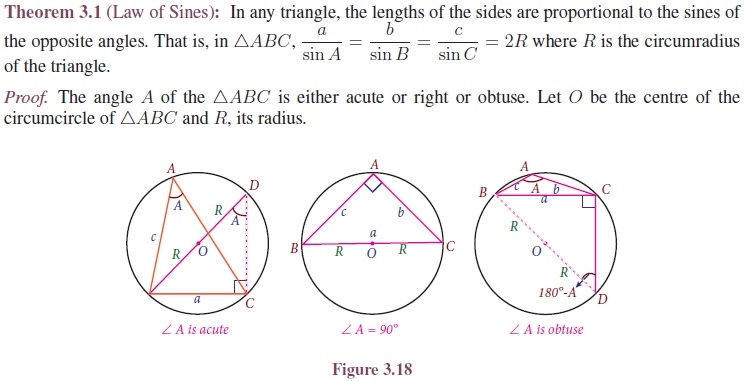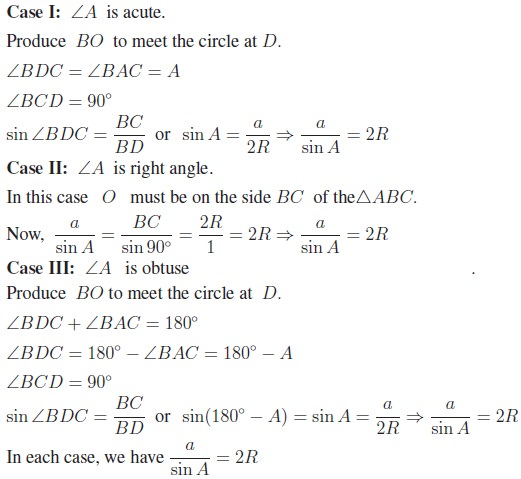Home | | Maths 11th std | The Law of Sines or Sine Formula

# The Law of Sines or Sine Formula

The Law of Sines is a relationship between the angles and the sides of a triangle.

The Law of Sines or Sine Formula

## 1. Law of Sines

The Law of Sines is a relationship between the angles and the sides of a triangle. While solving a triangle, the law of sines can be effectively used in the following situations:

i.            To find an angle if two sides and one angle which is not included, by them are given.

ii.            To find a side, if two angles and one side which is opposite to one of given angles, are given.'## 2. Law of Cosines

When two sides and included angle or the three sides of a triangle are given, the triangle cannot be solved using the sine formula. In such a situation, the law of cosines can be used to solve the triangle. Also, the Law of Cosines is used to derive a formula for finding the area of a triangle given two sides and the included angle.## 3. Projection Formula## 4. Area of the Triangle

We shall use some elements of an oblique triangle and the sine function to find the area of the triangle. Recall that area formula for   ŌłåABC is ┬Į bh  where b is the base and h is the height. For oblique triangle, we must find h before using the area formula.## 5. Half-Angle formulaTags : Definition, Formula, Solved Example Problems, Exercise | Mathematics , 11th Mathematics : UNIT 3 : Trigonometry
Study Material, Lecturing Notes, Assignment, Reference, Wiki description explanation, brief detail
11th Mathematics : UNIT 3 : Trigonometry : The Law of Sines or Sine Formula | Definition, Formula, Solved Example Problems, Exercise | Mathematics